Physical Interpretation of Noncommutative Algebraic Varieties | OMICS International
Journal of Physical Mathematics
All submissions of the EM system will be redirected to Online Manuscript Submission System. Authors are requested to submit articles directly to Online Manuscript Submission System of respective journal.

# Physical Interpretation of Noncommutative Algebraic Varieties

Siqveland A*

Faculty of Technology, Buskerud University College Kongsberg, Norway

*Corresponding Author:
Siqveland A
Faculty of Technology
Buskerud University College Kongsberg, Norway
Tel: +47 31 00 89 18
E-mail: [email protected]

Received Date: March 31, 2015; Accepted Date: April 11, 2015; Published Date: April 21, 2015

Citation: Siqveland A (2015) Physical Interpretation of Noncommutative Algebraic Varieties. J Phys Math 6:132. doi:10.4172/2090-0902.1000132

Copyright: © 2015 Siqveland A. This is an open-access article distributed under the terms of the Creative Commons Attribution License, which permits unrestricted use, distribution, and reproduction in any medium, provided the original author and source are credited.

Visit for more related articles at Journal of Physical Mathematics

#### Abstract

The theory of algebraic varieties gives an algebraic interpretation of differential geometry, thus of our physical world. To treat, among other physical properties, the theory of entanglement, we need to generalize the space parametrizing the objects of physics. We do this by introducing noncommutative varieties.

#### Keywords

Noncommutative algebra; Differential geometry; Matrix polynomial algebras

#### Introduction

Differential geometry is simplified by applying algebraic geometry. Then all holomorphic functions are interpreted as polynomials, and completed to power series. We have used infinitesimal methods, or rather deformation theory of modules, to construct varieties as moduli of the closed points. Eriksen  and Laudal  for a precise treatment. Then a generalization to deformation theory over commutative rings to noncommutative, have made the definition of noncommutative varieties possible.

In this short text, we show how our results can be stated without mentioning deformation theory, and we show one way of interpreting noncommutative varieties of matrix polynomial algebras.

Finally, we show how our notion of entanglement gives a kind of smoothing of a singularity. In articles to come, we will show how these varieties can (and must) be constructed using deformation theory.

#### Matrix Polynomial Algebras

Definition 1

Let M ∈ Mr be a matrix with natural numbers mij as entries, and let R be a commutative ring. We then let R[M] be the R-algebra generated by the matrices (paths) tij (lij ), 1 ≤ lij ≤ mij, 1 ≤ i, j ≤ r. Alternatively R[M ] =TR,(VM) the tensor algebra over Rr` of the module generated by the tij (lij) given by matrix multiplication.

Example 1. We let r=2 and M=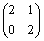.Then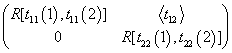,

the R2=R · id(2)-algebra generated by the matrix generators.

We should notice that in general algebraic theory, there is no reason why the algebras on the diagonal, that is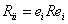, should be commutative. This is the reason that we use the square brackets in the notation. The general, not necessarily commutative, polynomial algebra over R with M variables should then be named R (M) .

For the rest of this text, we will let R=k, an algebraically closed field of characteristic 0. And of course, because we are giving the physical interpretation, we think of k as the field of complex numbers.

#### The Non Commutative Affine Space

We put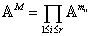For a matrix polynomial, that is an element f=(fij) ∈ k[M ], and apoint P = (p1, ...., pr ) ∈ kr , we let f (P ) = (f11(p1), . . . , frr (pr )). Then as usual, we let the complements of the algebras sets be the topology of AM.

Definition 2

Let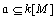be a two-sided ideal. Then the set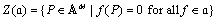is called an algebraic set, or a Zariski closed set.

The resulting topology is obviously the product topology of the Zariski topology, as the entries off the diagonal doesn’t influence the state. As we will see, these variables are present to make the dynamics in the space. Each of the com- mutative affine spaces in the product corresponds to the ordinary Euclidean space.

As in the commutative situation, we let the coordinate ring of an algebraic set Z(a) be A[M] / a (we assume that algebraic sets are reduced). The ring of regular functions is the most problematic one to define in the noncommutative case. This is where the dynamics, or entanglement, comes into the picture. We need to translate the deformation theory to the concept of a semi-local ring.

Lemma 1: The simple one-dimensional k[M]-modules corresponds to the maximal ideals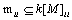. Thus there is a one-to-one correspondence between point in AM and tuples of maximal ideals with one-dimensional quotient.

Definition 3

Let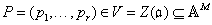. We define the semi- local ring of P in V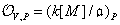a where the notation RP for a matrix algebra R means that each Rii is localized in pi

Example 2: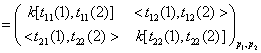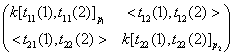Then the above mentioned transformation of the deformation theory is that a regular function is a global function that is regular in every point:

Definition 4

Let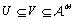M be an open subset of the affine variety V. Then the ring of regular functions over U is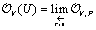#### The Dynamics in Noncommutative Affine Varieties

The dynamics in any space is given by the structure of the tangents. This is because the tangent space is the space generated by the tangent directions, which are the directions in which the actual geometry allows dynamics. Thus the tangent space tells what kind of changes in state that are possible. Let us recall the commutative situation, equivalently the differential geometric one: Consider a derivation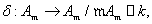satisfying the Leibniz ruleδ (ab) = aδ (b) +δ (a)b . This induces a k-linear morphism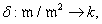which is an element in the dual space (m /m 2 )∨ . Thus in the ordinary (commutative) setting, the directions of change is the basis element in the tangent space (m /m2 )∨ . When we let one point actually be r points, we have to generalize slightly.

Let R be a quotient of a matrix polynomial algebra, and let Vi and Vj be two simple one-dimensional left R-modules. Then Homk ( Vi , Vj ) is a left-right bi module by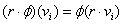and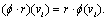Definition 5: The 1-radical of R is defined as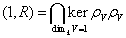where ρV: R → Endk (V) is the structure morphism of V .

Of course, this indicates that we should really work with the Jacobson radical, taking all simple modules into account. This applied theory is generalized in work to be published in the near future. For the rest of this section, we use the word radical for the 1-radical, and we use the notation m=I(1, R). Then we define the tangent space in this situation as:

Definition 6: The tangent space of the affine variety V in the point p= (p1,..pr) is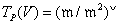where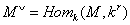for any A(V)-module M .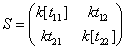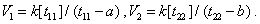First, we compute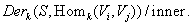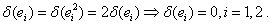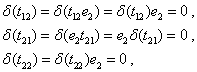and finally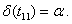As all inner derivations are zero (easily seen from the computation above, in particular because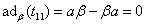we find that Derk (V1, V1)/Inner is generated by the derivation sending t11 to α, and all other generators to 0.

(1, 2):

For ∈ Derk (S, Homk (V1, V2)) things are slightly different.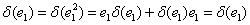(e ) , that is, the above trick doesn’t work quite the same way. However, as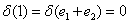for every derivation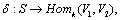we find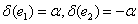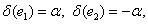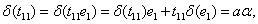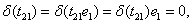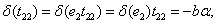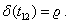So a general derivation can be written, the ∗ denoting the dual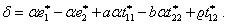For the inner derivations, we compute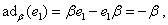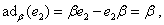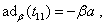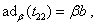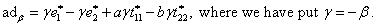So as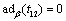and there are no conditions on δ(t12), we Derk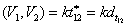The cases (2, 1) and (2, 2) are exactly similar.

Generalizing the computation in the above example, we have proved the following:

Lemma 2: Let S be a general free r×r matrix polynomial algebra, and let Vi=Vii(pii) be the point pii in entry i, i. Then the tangent space from Vi to Vj is Derk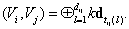#### Entanglement: Blowing Up a Singularity

For a precise treatment of the deformation theory leading to this examples, see . We consider a coincidently chosen singularity, the E6-singularity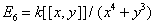This is a singularity because the tangent space is of dimension d>1 which is too big. So we need to make space for the entanglement, that is the interrelations. Let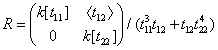For a point P = (α1, α2) in this space, we compute the tangent space in this point by applying Lemma 2.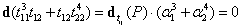Suggest that the points are pairwise identified, and with an extra tangent from zero to zero. This justifies that we can call this a blowup of the singularity.

Of course, it would be more obvious if we, in the matrix above, localized in origo, then the identification is superfluous. Anyway, the interpretation must be taken by care.

Also, there is a long way to go to prove that the physicist’s notion of entanglement has anything to do with this momentum between different points. Our main achievement is to give an example on how extra space for a tangent direction can be given.

#### References

Select your language of interest to view the total content in your interested language

### Article Usage

• Total views: 12250
• [From(publication date):
April-2015 - Nov 20, 2019]
• Breakdown by view type
• HTML page views : 8459Can't read the image? click here to refresh